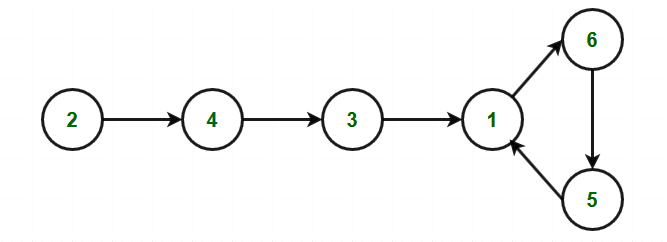# Find any one of the multiple repeating elements in read only array | Set 2

Given a read-only array arr[] of size N + 1, find one of the multiple repeating elements in the array where the array contains integers only between 1 and N.
Note: Read-only array means that the contents of the array can’t be modified.

Examples:

Input: N = 5, arr[] = {1, 1, 2, 3, 5, 4}
Output: 1
Explanation:
1 is the only number repeated in the array.

Input: N = 10, arr[] = {10, 1, 2, 3, 5, 4, 9, 8, 5, 6, 4}
Output: 5
Explanation:
5 is the one of the number repeated in the array.

## Recommended: Please try your approach on {IDE} first, before moving on to the solution.

In the previous post, we have discussed the same article with a space complexity O(N) and O(sqrt(N)).

Approach: This approach is based on Floyd’s Tortoise and Hare Algorithm (Cycle Detection Algorithm).

1. Use the function f(x) = arr[x] to construct the sequence:

arr, arr[arr], arr[arr[arr]], arr[arr[arr[arr]]] …….

2. Each new element in the sequence is an element in arr[] at the index of the previous element.
3. Starting from x = arr, it will produce a linked list with a cycle.
4. The cycle appears because arr[] contains duplicate elements(at least one). The duplicate value is an entrance to the cycle. Given below is an example to show how cycle exists:
For Example: Let the array arr[] = {2, 6, 4, 1, 3, 1, 5}

Starting from index 0, the traversal looks as follows:

arr = 2 –> arr = 4 –> arr = 3 –> arr = 1 –> arr = 6 –> arr = 5 –> arr = 1.

The sequence forms cycle as shown below:5. Algorithm consists of two parts and uses two pointers, usually called tortoise and hare.
• hare = arr[arr[hare]] is twice as fast as tortoise = arr[tortoise].
• Since the hare goes fast, it would be the first one who enters the cycle and starts to run around the cycle.
• At some point, the tortoise enters the cycle as well, and since it’s moving slower the hare catches the tortoise up at some intersection point.
• Note that the intersection point is not the cycle entrance in the general case, but the two intersect at somewhere middle in cycle.
• Move tortoise to the starting point of sequence and hare remains within cycle and both move with the same speed i.e. tortoise = arr[tortoise] and hare = arr[hare]. Now they intersect at duplicate element.

Below is the implementation of the above approach:

## C++

 `// C++ code for the above approach ` `#include ` `using` `namespace` `std; ` ` `  `// Function to find the duplicate ` `// value in the given array arr[] ` `void` `findDuplicate(``int` `arr[]) ` `{ ` ` `  `    ``// Initialise variables ` `    ``int` `tortoise = arr; ` `    ``int` `hare = arr; ` ` `  `    ``// Loop till we find the ` `    ``// duplicate element ` `    ``while` `(1) { ` ` `  `        ``tortoise = arr[tortoise]; ` ` `  `        ``// Hare moves with twice ` `        ``// the speed of tortoise ` `        ``hare = arr[arr[hare]]; ` `        ``if` `(tortoise == hare) ` `            ``break``; ` `    ``} ` ` `  `    ``tortoise = arr; ` ` `  `    ``// Loop to get start point ` `    ``// of the cycle as start ` `    ``// point will be the duplicate ` `    ``// element ` `    ``while` `(tortoise != hare) { ` `        ``tortoise = arr[tortoise]; ` `        ``hare = arr[hare]; ` `    ``} ` ` `  `    ``// Print the duplicate element ` `    ``cout << tortoise; ` `} ` ` `  `// Driver Code ` `int` `main() ` `{ ` `    ``// Given array ` `    ``int` `arr[] = { 2, 6, 4, 1, 3, 1, 5 }; ` ` `  `    ``// Function Call ` `    ``findDuplicate(arr); ` ` `  `    ``return` `0; ` `} `

## Python3

 `# Python3 program for the above approach  ` ` `  `# Function to find the duplicate  ` `# value in the given array arr[]  ` `def` `findDuplicate(arr):  ` ` `  `    ``# Initialise variables  ` `    ``tortoise ``=` `arr[``0``]  ` `    ``hare ``=` `arr[``0``] ` ` `  `    ``# Loop till we find the  ` `    ``# duplicate element  ` `    ``while` `(``1``):  ` ` `  `        ``tortoise ``=` `arr[tortoise] ` ` `  `        ``# Hare moves with twice  ` `        ``# the speed of tortoise  ` `        ``hare ``=` `arr[arr[hare]]  ` `        ``if` `(tortoise ``=``=` `hare):  ` `            ``break` ` `  `    ``tortoise ``=` `arr[``0``]  ` ` `  `    ``# Loop to get start point  ` `    ``# of the cycle as start  ` `    ``# point will be the duplicate  ` `    ``# element  ` `    ``while` `(tortoise !``=` `hare): ` `        ``tortoise ``=` `arr[tortoise]  ` `        ``hare ``=` `arr[hare] ` ` `  `    ``# Print the duplicate element  ` `    ``print` `(tortoise)  ` ` `  `# Driver Code  ` ` `  `# Given array  ` `arr ``=` `[ ``2``, ``6``, ``4``, ``1``, ``3``, ``1``, ``5` `] ` ` `  `# Function Call  ` `findDuplicate(arr) ` ` `  `# This code is contributed by PratikBasu `

Output:

```1
```

Time Complexity: O(N)
Auxiliary Space: O(1)

Don’t stop now and take your learning to the next level. Learn all the important concepts of Data Structures and Algorithms with the help of the most trusted course: DSA Self Paced. Become industry ready at a student-friendly price.

My Personal Notes arrow_drop_upCheck out this Author's contributed articles.

If you like GeeksforGeeks and would like to contribute, you can also write an article using contribute.geeksforgeeks.org or mail your article to contribute@geeksforgeeks.org. See your article appearing on the GeeksforGeeks main page and help other Geeks.

Please Improve this article if you find anything incorrect by clicking on the "Improve Article" button below.

Improved By : PratikBasu